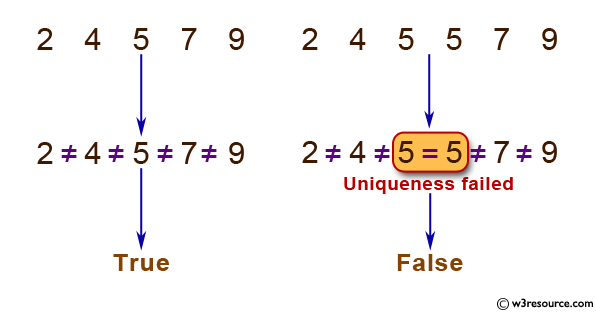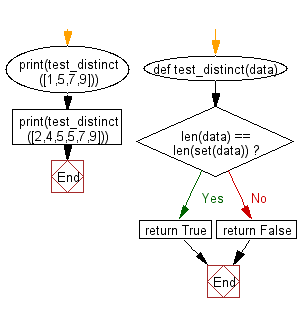﻿ Python: Function that takes a sequence of numbers and determines whether all are different from each other - w3resource

# Python: Function that takes a sequence of numbers and determines whether all are different from each other

## Python Basic - 1: Exercise-1 with Solution

Write a Python function that takes a sequence of numbers and determines whether all the numbers are different from each other.

Pictorial Presentation:Sample Solution:

Python Code :

``````def test_distinct(data):
if len(data) == len(set(data)):
return True
else:
return False;
print(test_distinct([1,5,7,9]))
print(test_distinct([2,4,5,5,7,9]))
``````

Sample Output:

```True
False
```

Flowchart:Python Code Editor :

Have another way to solve this solution? Contribute your code (and comments) through Disqus.

What is the difficulty level of this exercise?

Test your Programming skills with w3resource's quiz.

﻿